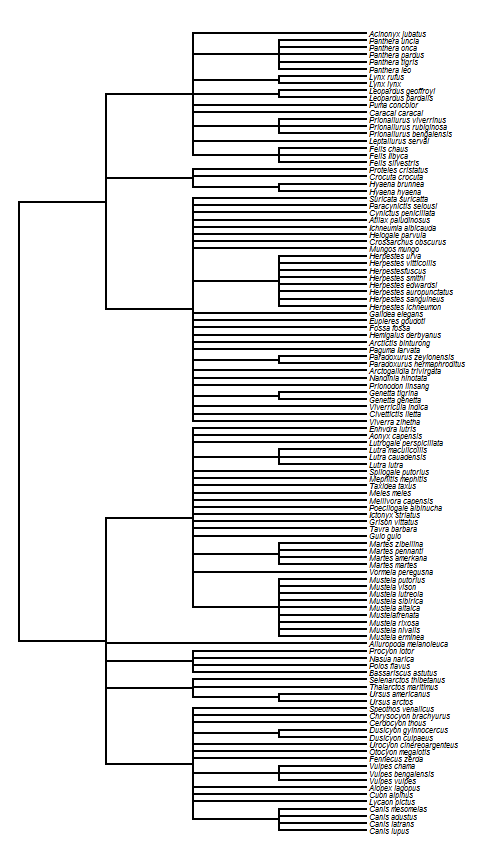## Tuesday, March 29, 2016

### `as.phylo.formula` in which a given taxonomic level is found at a constant depth

Unfortunately, the R-sig-phylo archive was recently made private due to some issues with spammers. This means that the links in several prior phytools blog posts may be dead. Sorry! If you encounter this issue and it is affecting your research, please let me know. I can try to update the link or supply information on how to find the archived messages on the searchable archive, which is not private.

The one post that I didn't realize was popular until the archive went down was a very simple modification that I made to the ape function `as.phylo.formula` that I described in a phytools blog post here. In this modification, divergences of a certain taxonomic level (e.g., family) are all forced to have the same depth.

For the record, here is the code:

``````## as.phylo.formula from ape written by Julien Dutheil
## (julien.duth...@univ-montp2.fr)
## modified by Liam Revell (liam.rev...@umb.edu)
## depends on ape & phytools
as.phylo.formula<-function (x, data = parent.frame(), ...){
err<-"Formula must be of the kind \"~A1/A2/.../An\"."
if(length(x)!=2)
stop(err)
if(x[]!="~")
stop(err)
f<-x[]
taxo<-list()
while(length(f)==3){
if(f[]!="/")
stop(err)
if(!is.factor(data[[deparse(f[])]]))
stop(paste("Variable",deparse(f[]),
"must be a factor."))
taxo[[deparse(f[])]]<-data[[deparse(f[])]]
if(length(f)>1)
f<-f[]
}
if(!is.factor(data[[deparse(f)]]))
stop(paste("Variable",deparse(f),"must be a factor."))
taxo[[deparse(f)]]<-data[[deparse(f)]]
taxo.data<-as.data.frame(taxo)
leaves.names<-as.character(taxo.data[,1])
taxo.data[,1]<-1:nrow(taxo.data)
f.rec<-function(subtaxo){
u<-ncol(subtaxo)
levels<-unique(subtaxo[,u])
if(u==1){
if(length(levels)!=nrow(subtaxo))
warning("Error, leaves names are not unique.")
return(as.character(subtaxo[,1]))
}
t<-character(length(levels))
for(l in 1:length(levels)){
x<-f.rec(subtaxo[subtaxo[,u]==levels[l],][1:(u-1)])
t[l]<-paste("(",paste(x,collapse=","),")",sep="")
}
return(t)
}
string<-paste("(",paste(f.rec(taxo.data),collapse=","),
");",sep="")
## so that singles will be read without error
phy\$edge.length<-rep(1,nrow(phy\$edge))
phy<-collapse.singles(phy)
phy\$tip.label<-leaves.names[as.numeric(phy\$tip.label)]
return(phy)
}
``````

And here's a demo of it's use:

``````library(phytools)
data(carnivora)
t1<-as.phylo.formula(~SuperFamily/Family/Genus/Species,
data=carnivora)
plotTree(t1,fsize=0.7,ftype="i")
``````You can try it with & without loading the modified function version, above, to see the difference.